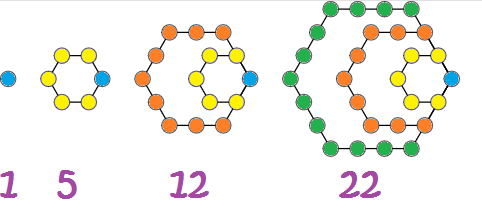# Definition of Pentagonal NumberA pentagonal number is a number that can be drawn using a pattern of dots in the shape of a pentagon, like the ones in the diagram.

The first six pentagonal numbers are $1,5,12,22,35$ and $51$.

### Description

The aim of this dictionary is to provide definitions to common mathematical terms. Students learn a new math skill every week at school, sometimes just before they start a new skill, if they want to look at what a specific term means, this is where this dictionary will become handy and a go-to guide for a student.

### Audience

Year 1 to Year 12 students

### Learning Objectives

Learn common math terms starting with letter P

Author: Subject Coach
You must be logged in as Student to ask a Question.